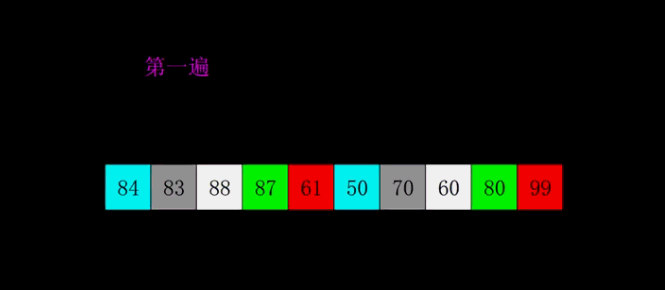# 希尔排序

#### 基本思想¶

• 将待排序数组按照一定的间隔分为多个子数组，每组分别进行插入排序。这里按照间隔分组指的不是取连续的一段数组，而是每跳跃一定间隔取一个值组成一组；
• 逐渐缩小间隔进行下一轮排序；
• 最后一轮时，取间隔为 $$1$$，也就相当于直接使用插入排序。但这时经过前面的「宏观调控」，数组已经基本有序了，所以此时的插入排序只需进行少量交换便可完成。• 定义增量序列 *$$D_m > D_{m-1} > D_{m-2} > ... > D_1 = 1$$
• 对每个 $$D_k$$ 进行 「$$D_k$$​ 间隔排序」。

#### 代码实现¶##### 基础版本¶
class Solution {
public:
vector<int> shellSortBasic(vector<int>& nums) {
for (int gap = nums.size() / 2; gap >= 1; gap /= 2) {
for (int start = 0; start < gap; start++) {
for (int current = start + gap; current < nums.size(); current += gap) {
int currentNum = nums[current];
int pre = current - gap;
while (pre >= 0 && currentNum < nums[pre]) {
nums[pre + gap] = nums[pre];
pre -= gap;
}
nums[pre + gap] = currentNum;
}
}
}
return nums;
}
};

##### 优化版本¶
class Solution {
public:
vector<int> shellSortOptimized(vector<int>& nums) {
for (int gap = nums.size() / 2; gap >= 1; gap /= 2) {
for (int i = gap; i < nums.size(); i++) {
int num = nums[i];
int pre = i - gap;
while (pre >= 0 && num < nums[pre]) {
nums[pre + gap] = nums[pre];
pre -= gap;
}
nums[pre + gap] = num;
}
}
return nums;
}
};


#### 增量序列的选择¶

• Hibbard 增量序列：$$D_k = 2^k - 1$$，也就是 $${1, 3, 7, 15, ...}$$。数学界猜想它最坏的时间复杂度为 $$O(n^{3/2})$$，平均时间复杂度为 $$O(n^{5/4})$$
• Knuth 增量序列：$$D_1 = 1; D_{k+1} = 3 * D_k + 1$$，也就是 $${1, 4, 13, 40, ...}$$，数学界猜想它的平均时间复杂度为 $$O(n^{3/2})$$
• Sedgewick 增量序列： $${1, 5, 19, 41, 109, ...}$$，这个序列的元素有的是通过 $$9 * 4^k - 9 * 2^k + 1$$ 计算出来的，有的是通过 $$4^k - 3 * 2^k + 1$$ 计算出来的。数学界猜想它最坏的时间复杂度为 $$O(n^{4/3})$$，平均时间复杂度为 $$O(n^{7/6})$$